[BACK]
 Intelligent Automation & Soft ComputingDOI:10.32604/iasc.2021.017607Article

Face Image Compression and Reconstruction Based on Improved PCA

1School of Computer and Software, Nanjing University of Information Science and Technology, Nanjing, 210044, China
2Jiangsu Key Laboratory of Data Science and Smart Software, Jinling Institute of Technology, Nanjing, 211169, China
3College of Information Engineering, Fuyang Normal University, Fuyang, 236041, China
4Department of Mathematics, Faculty of Science, New Valley University, El-Kharga, 72511, Egypt
*Corresponding Author: Yu Xue. Email: xueyu@nuist.edu.cn
Received: 04 February 2021; Accepted: 02 July 2021

Abstract: Face recognition technology has many usages in the real-world applications, and it has generated extensive interest in recent years. However, the amount of data in a digital image is growing explosively, taking up a lot of storage and transmission resources. There is a lot of redundancy in an image data representation. Thus, image compression has become a hot topic. The principal component analysis (PCA) can effectively remove the correlation of an image and condense the image information into a characteristic image with several main components. At the same time, it can restore different data images according to their principal components and meet the needs of image compression and reconstruction at diverse levels. This paper introduces an improved PCA algorithms. The covariance matrix, calculated according to a batch of training samples, is an approximation of the real covariance matrix. The matrix is relatively to the dimension of the covariance matrix, and the number of training samples is often too small. Therefore, it difficult to accurately obtain the covariance matrix. This improved PCA algorithm called 2DPCA can solve this problem effectively. By comparing it with several discrete PCA improvement algorithms, we show that the 2DPCA has a better dimensionality reduction effect. Compared with the PCA algorithm, the 2DPCA has a lower root-mean-square error under the constant noise condition.

Keywords: Image compression; PCA; feature extraction

1  Introduction

At present, the amount of digital image data is soaring, occupying a lot of storage space and apportioning increased transmission resources . Due to the high correlation of adjacent pixels, there is a lot of redundancy in image data representation. The principal component analysis (PCA) method can remove the correlation of the image data , and effectively compress the image information into several main components. At the same time, it can restore different data images according to their number of principal components, thus meet the needs of image compression and reconstruction at different levels. Moreover, PCA is often used for feature selection .

Among the active subspaces, the researchers’ top concern is the face image. It has been of a wide concern and deeply studied by the academic community. Feature extraction and dimension reduction are the key steps of face compression . However, there are many shortcomings in the PCA algorithm. The common PCA compression method cannot achieve good results due to external conditions such as change of facial expression and strong light. Another important factor to consider is the dimension of the pictures . Therefore, it is necessary to study an improved PCA algorithm, which can enhance the compression efficiency and ameliorates the accuracy of reconstruction . It should be noticed that self-adaptive parameter is a good direction to optimization PCA. The image compression and reconstruction can be used in drones . It should be noticed that self-adaptive parameter is a good direction to optimization PCA .

The main work of this paper is to study and analyzes the PCA algorithm for image compression and reconstruction. This paper focus on the study of PCA improved algorithm which includes 2DPCA, Mat PCA and Module PCA. The rest of the article is structured as follows: Section 2 introduces related work. Section 3 describes the PCA and improved PCA. Section 4 introduces the designs of experiment and analysis the experimental results. Finally, Section 5 provides the conclusions.

2  Related Work

PCA is also called principal component analysis. It is a statistical method that converts the original multiple variables into several new composite variables . These new variables are uncorrelated with each other and effectively to represent the information of the original variables. PCA can remove the correlation between image data, and condense the image information on the characteristic image which is several main components. The PCA is effectively to realize image compression. At the same time, it can recover different data image according to the number of principal components which are meet the needs of image compression and reconstruction at diverse levels. To conduct data analysis through deep learning [15,16]. The PCA can be used to preprocess multi-objective optimization algorithms . The basic PCA image compression algorithm can achieve ideal compression ratio, but this method does not have a good standard for the selection of the number of retained features. The signal-to-noise ratio is very low, and the non-linear or non-stationary image signals are not considered. At the same time, the algorithm is optimized by the evolutionary algorithm and deep learning [18,19].

3  Improved PCA Algorithms

The pixel represents redundant information on the face image. It can be used to subtract the predicted value IP from the actual value I , which obtain the difference value ΔI , and value ΔI is known as the prediction error. Finally, the prediction error is only compressed and encoded. Since the predicted value of each pixel only uses the previously encoded pixel, this coding process is also said to be causal. The decoding process based on causal encoding is shown in Fig. 1.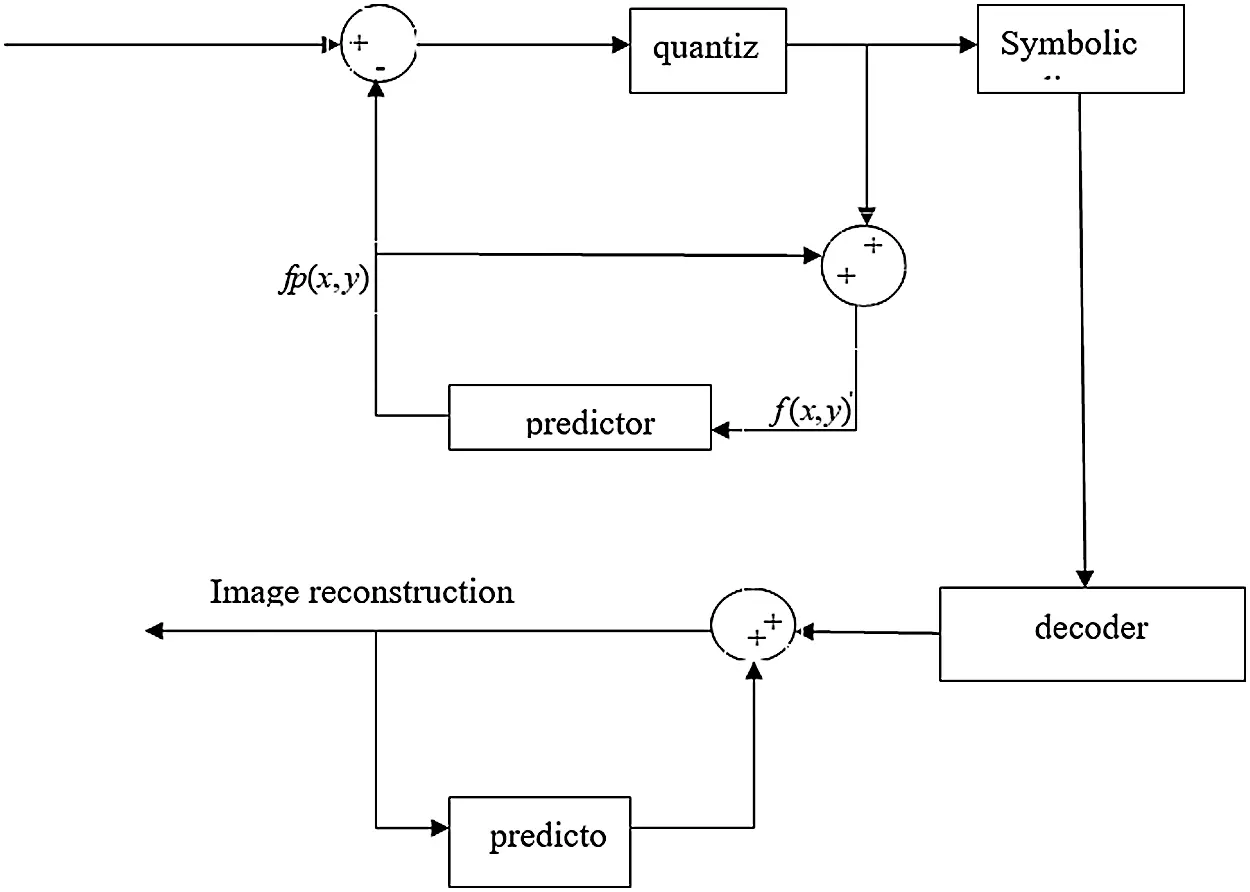Figure 1: A classical predictive based compression system

Another way of image compression is transformation. In the process of transformation, the image is first obtained by some transforming (linear or nonlinear), and then which quantize these coefficients to obtain the compressed image. At the end of decoding, the encoded coefficients are quantized inversely, and the actual image are produced by inverse transformation. A typical transformation based on compression system is shown in Fig. 2.Figure 2: A typical transformation-based compression system

Both forecast code and conversion code have their own advantages. The former one is relatively simple to implement, and the algorithm itself is adaptive to the original information of the image. The latter one generally has a higher compression ratio, but the cost is the complexity of transformation calculation, which also makes the implementation more complex. The evaluation method of image compression is usually divided into two aspects, compression performance and compression image quality. Compression performance is usually measured by compression ratio CR or relative data redundancy R , which is defined as the ratio between the total amount of original data b and the total amount of compressed data b . Relative data redundancy R is defined as the percentage of the reduced amount of compressed data relative to the original amount of data:

CR=bb (1)

R=Δbb=bbb=11CR (2)

bpp=bpixels (3)

erms=1MNx=0M1y=0N1[I^(x,y)I(x,y)]2 (4)

SNR=x=0M1y=0N1I^(x,y)2x=0M1y=0N1[I^(x,y)I(x,y)]2 (5)

PSNR=10log(2n1)2MSE (6)

The quality of the compressed image can be evaluated either subjectively or objectively. Among them, common objective quality evaluation methods include root mean square error, SNR (signal to noise ratio) and PSNR (peak signal to noise ratio).

The I(x,y) and I^(x,y) represent decompressed image and original image respectively. In PSNR calculation formula, N is equal to the bits per pixel, usually 8 bits, and MSE represents the root mean square error. Although the factual quality assessment method is convenient and feasible, it can’t really reflect people’s subjective feelings towards the image, so the subjective quality assessment is more accurate. In this paper, the root mean square error is used primarily to evaluate the quality of image reconstruction.

3.1 PCA

K-L transformation is one of the main processes of the PCA method. It is necessary to use K-L transformation to realize facial image compression and reconstruction. The K-L transformation method is classical and easy to implement. The basic PCA method first selects some image as training image before facial image compression. Assuming that the image to be trained has a size of N2×N2 , the pixels of all its columns can be joined end to end. In this way, each image can be stretched into a column vector of length N2 which can be viewed as a point in N2 dimensional space. Because the training image has a lot of similarities between each other, the vector on the shearing section is also different. The vector in high-dimensional space distribution is not random or chaotic, principal component analysis has the very strong correlation between each other, which uses a low-dimensional subspace to describe image. Assuming that the spatial description of these training image sets contains m sets of images, let Xi,i{1,2,3,,m} to be the image vector of i training sample, and x=[x1,x2,,xm] , u is the average image vector of all training sample images, namely:

u=1Mi=1Mxi (7)

PCA requires the population dispersion matrix of the training sample set, which named the covariance matrix:

=E[(xu)(xu)T] (8)

It is a matrix with dimension N2×N2 and the principal component analysis method needs to calculate its eigenvalues and orthogonal normalized eigenvectors. Since N2 will be very large in practical application, it is very difficult to directly calculate the formula. For this reason, SVD decomposition can well solve this problem.

3.1.1 SVD Decomposition

SVD decomposition is a common method to deal with matrices with high dimensions. SVD decomposition can effectively decompose high-dimensional matrices into low-dimensional space. Through SVD decomposition, we can solve the eigenvalues of the high-dimensional matrix easily. The following is the exact theory related to SVD decomposition.

If A is n×r dimensional matrix of rank R, then there are two orthogonal matrices:

U=(u1,u2,,ur)n×r (9)

V=(v1,v2,,vr)n×r (10)

Λ=dig(λ1,λ2,,λr)n×r (11)

A=UA12VT (12)

where, λi(i=1,2,,r) is the non-zero eigenvalue of matrix AAT and ATA , ui and vi are eigenvectors of AAT and ATA . The above decomposition becomes the Singular Value Decomposition (SVD) of matrix A, and the λi can be expressed as:

=1Mi=1M(xiu)(xiu)T=1MXXT (13)

Therefore, construct the matrix:

R=XTXM×M (14)

It is easy to find its eigenvalue λi and corresponding orthogonal normalized eigenvector vi(i=1,2,,M) . From the above inference, the orthogonal normalized eigenvector is:

ui=1λiXvi,i=1,2,,M (15)

Arranging the eigenvalues from large to small: λ1λ2λM, their corresponding eigenvector is ui , in this way, each face image can be projected into a subspace of { u1,u2,,uM } spans. Therefore, each face image is commensurate with a point in the subspace. Similarly, any point in the subspace corresponds to an image. With such { u1,u2,,uM } span subspace, any face image can be projected onto it and a set of coordinate coefficients are obtained, which show the position of the image in the subspace. In other words, any face image can be represented as a linear combination of { u1,u2,,uM } and its weighted coefficient is the expansion coefficient of K-L transformation, which can also be called the algebraic feature of the image.

For any face image f to be compressed, its coefficient vector can be obtained by projecting it into the feature subspace:

y=UT(fu),U=(u1,u2,,uM) (16)

The resulting coefficient vector can be thought as a compression. Since the coefficient vector dimension m is usually much less than f, thus it saves storage space greatly. It can be transformed back from u :

f=Uy+u (17)

3.2 Improved Algorithm

Basic principally component analysis method has some disadvantages. When the face image illumination position changes, basic PCA cannot capture these changes effectively. Studies have shown that the basic PCA can capture some of the most simply consistency between image hardly, unless the information is included in training image. In addition, the basic PCA will stretch the pixels of the image in some way (usually the first place of each column is connected) into a vector with high dimension. When the image size is bigger, the vector dimension after stretching will be very prominent, not to mention the covariance matrix between the training image. Although the SVD decomposition can be utilized for approximating the feature image, which avoid the emergence of large covariance matrix, it is not accurate in many cases. Due to the deficiency in the PCA method, an improved method, named 2DPCA is proposed in this paper.

Let’s X present a n dimensional normalized column vector. The 2DPCA algorithm take the image A ( A matrix of ×n ) according to the formula:

Y=AX (18)

we going to project it onto X . Thus, we get M dimensional projection vector Y , which is called the projection eigenvector of image A . Finding a good projection direction X in the 2DPCA method is a key step, and the strength of the projection vector X can be determined by the dispersion degree after training samples are projected on it. The higher the dispersion of the sample after projection, the better the projection direction X .

Through the study we know that we can use the trace of the covariance of the projected vector to describe the dispersion degree of the projected sample. That is:

J(X)=tr(Sx) (19)

where, Sx represents the covariance of the vector after training samples are projected on it; tr(Sx) is the trace of Sx .

The physical meaning of the maximization equation is to find a projection direction that maximizes the dispersion between the vectors after all training samples are projected on it. The covariance matrix of Sx can be expressed as:

Sx=E(YE(Y))(YE(Y))T (20)

=E[AXE(AX)][AXE(AX)]T=E[(AE(A))X][AE(A))X]Ttr(Sx)=XT[E(AE(A))T(AEA)]X (21)

Gt=E[(AE(A))T(AE(A))] (22)

The matrix Gt is called the image covariance matrix. It’s easy to know by definition that Gt is a nonnegative definite matrix for ×n . The training sample image can be used to directly calculate Gt . Suppose there are M training image samples, m×n matrix Aj(j=1,2,3,,m) represents the j training image, and A¯ represents the average image of all training samples.

A¯=1Mj=1MAj (23)

Gt=1Mj=1M(AjA¯)T(AjA¯) (24)

J(X)=XTGtX (25)

Eq. (25) is generalized criterion. The normalized vector Xopt that maximizes J(X) is called the optimal projection axis. According to literature, we can know that Xopt is the eigenvector corresponding to the maximum eigenvalue of Gt . Generally speaking, it’s not enough to have one optimal projection direction. It is necessary to find a series of projection directions, the set of projection directions is {X1,X2,,Xd} , which satisfies the principle of maximizing the J(X) :

{{X1,X2,,Xd}=argmaxJ(X)XiTXj=0,ij,i,j=1,2,,d (26)

In fact, in the projection direction set {X1,X2,,Xd} , satisfying the above principle is the orthogonal eigenvectors which corresponds to the first maximum eigenvalue of Gt .

4  Experimental Results

This section mainly summarizes the experimental results of the above algorithms, including the degree of image compression and the size of the root mean square error in image reconstruction. This paper mainly assesses the quality of several algorithms based on the size of the reconstruction error.

From Tab. 1, we can see that PCA algorithm can achieve an image compression ratio of about 3, and the image of the ORL database can have a very stable effect. As shown in Tab. 2, when noise is added, there will be no difference in compression effect.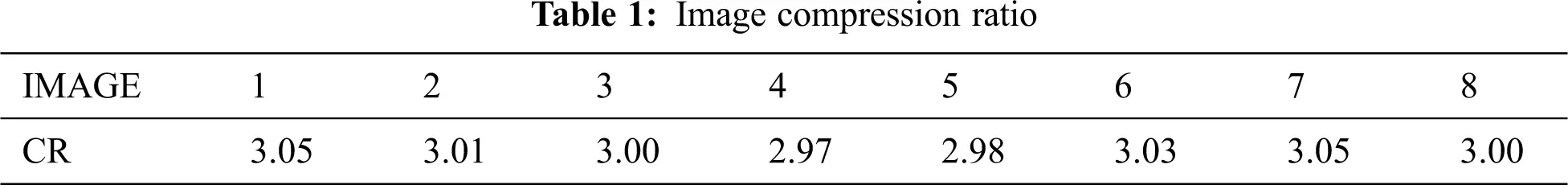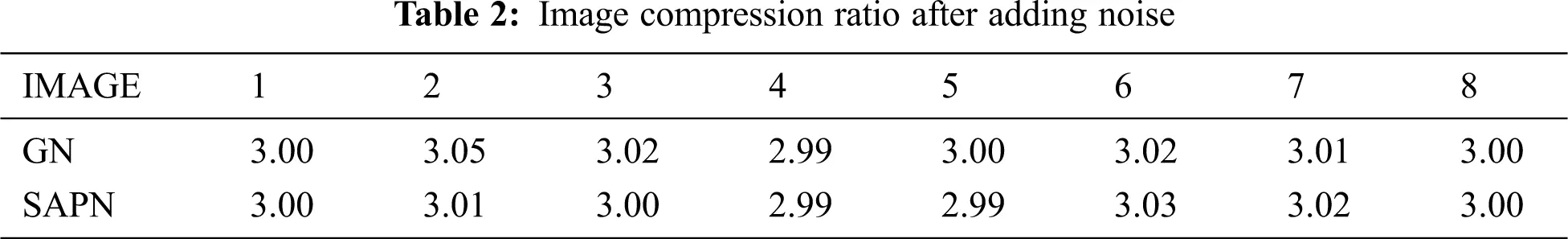From the above table, it shows that no matter what noise is added to PCA image compression. It has no significant influence on the image compression result. But adding noise will have a huge impact on image reconstruction. Tab. 3 shows the mean square deviation value of image reconstruction data adds noise.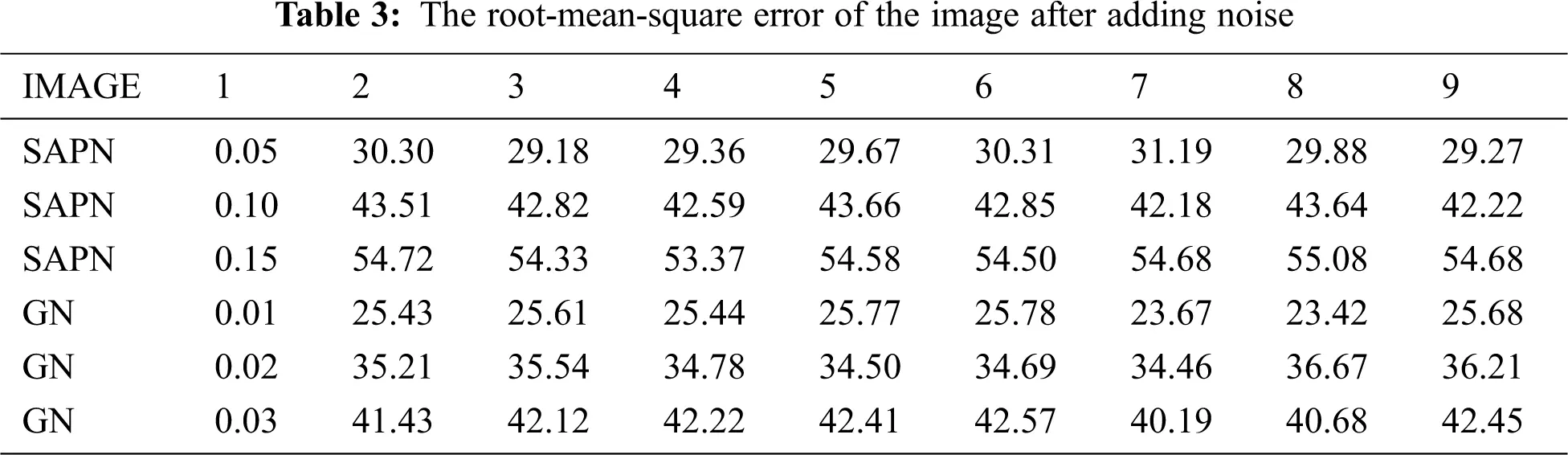In Tab. 4, the mean value of Gaussian noise is all 0 by default. From Tab. 4, we can see that the 2DPCA algorithm also have better results when processing noisy image. Compared with PCA algorithm, 2DPCA algorithm has lower root-mean-square error under the same noise condition. From Tab. 5, we can see that compared with Mat PCA, 2DPCA also has lower root-mean-square error under the same noise.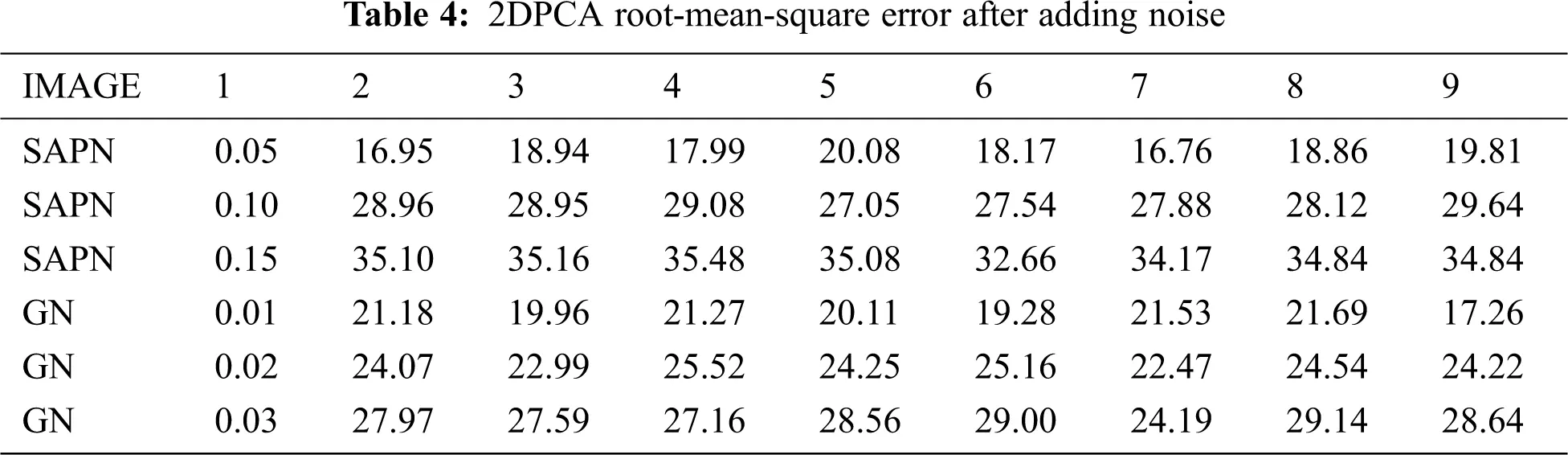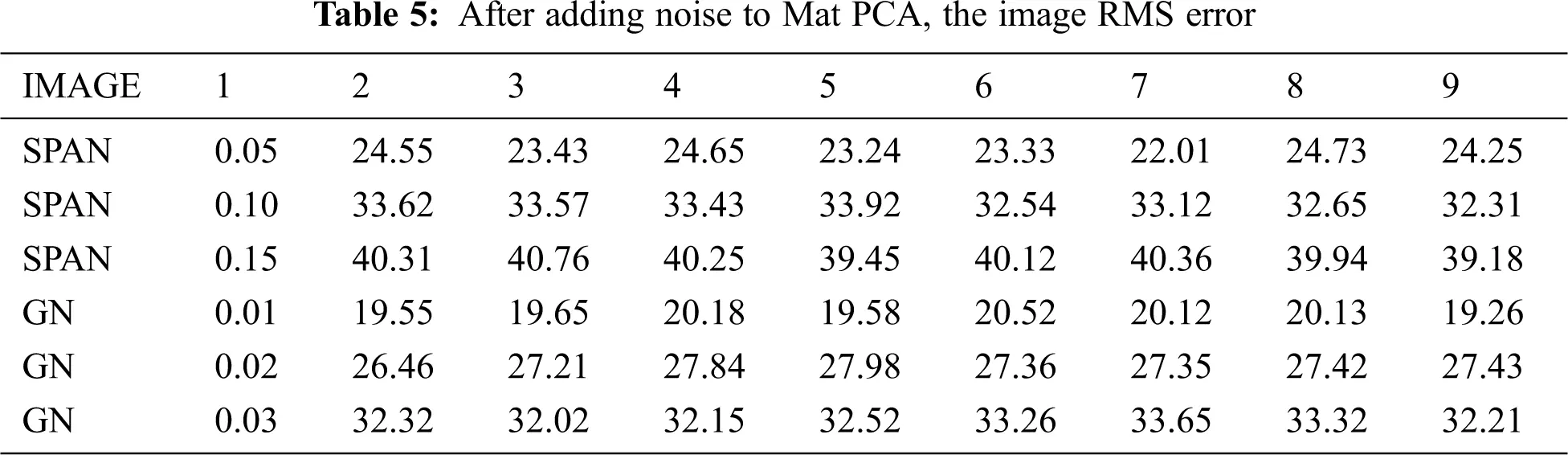5  Conclusion

This article provides an image reconstruction and compression algorithm based on principal component analysis and its improved algorithm. PCA is effective to reduce the dimension of data and minimize the error between the extracted components and the original data, so it can be used to data compression and feature extraction. Especially with the development of multimedia image data information technology, abundant image media contains a lot of information. In order to store and transmit these image data effectively, more and more attention is being paid to image compression technology. The image compression and reconstruction based on PCA and its improved algorithm is proposed in this paper. The experimental results demonstrate that the implementation method is simple. It can realize image compression effectively and restore different data images according to the number of principal components. It also satisfies the needs of different levels of image compression and reconstruction.

Funding Statement: This work was partially supported by the National Natural Science Foundation of China (61876089, 61876185, 61902281, 61375121), the Opening Project of Jiangsu Key Laboratory of Data Science and Smart Software (No. 2019DS301), the Science and Technology Program of Jiangsu Province Construction System (2020JH08), and the Priority Academic Program Development of Jiangsu Higher Education Institutions.

Conflicts of Interest: The authors declare that they have no conflicts of interest to report regarding the present study.

## References

1. D. Tsiotas and S. Polyzos, “The complexity in the study of spatial networks: An epistemological approach,” Networks & Spatial Economics, vol. 18, no. 1, pp. 1–32, 2018.
2. B. Y. Cai and J. Xu, “Digital image compression based on BEMD and PCA,” Computer Engineering and Applications, vol. 22, no. 1, pp. 335–337, 2011.
3. Y. Zhang, X. F. Song and D. W. Gong, “A return-cost-based binary firefly algorithm for feature selection,” Information Sciences, vol. 11, no. 2, pp. 418–419, 2017.
4. Y. Zhang, D. W. Gong, Y. Hu and W. Q. Zhang, “Feature selection algorithm based on bare bones particle swarm optimization,” Neurocomputing, vol. 14, no. 8, pp. 150–157, 2015.
5. Y. Zhang, D. W. Gong and J. Cheng, “Multi-objective particle swarm optimization approach for cost-based feature selection in classification,” IEEE-ACM Transactions on Computational Biology and Bioinformatics, vol. 14, no. 1, pp. 64–75, 2017.
6. M. L. Zheng, P. Z. Zhang and W. W. Guo, “Super resolution reconstruction of face image based on learning,” Computer Engineering and Applications, vol. 20, no. 5, pp. 122–130, 2013.
7. H. Jiang, “Image compression and reconstruction of principal component analysis,” Electronic Design Engineering, vol. 20, no. 5, pp. 14–18, 2012.
8. D. Zheng, Z. Ran, Z. Liu, L. Li and L. Tian, “An efficient bar code image recognition algorithm for sorting system,” Computers Materials & Continua, vol. 64, no. 3, pp. 1885–1895, 2020.
9. D. Wu, Y. Liu, Z. Xu and W. Shang, “Design and development of unmanned surface vehicle for meteorological monitoring,” Intelligent Automation & Soft Computing, vol. 26, no. 5, pp. 1123–1138, 2020.
10. Y. Xue, T. Tang, W. Pang and A. X. Liu, “Self-adaptive parameter and strategy based particle swarm optimization for large-scale feature selection problems with multiple classifiers,” Applied Soft Computing, vol. 88, no. 4, pp. 1–12, 2020.
11. Y. Xue, B. Xue and M. Zhang, “Self-adaptive particle swarm optimization for large-scale feature selection in classification,” ACM Transactions on Knowledge Discovery from Data, vol. 13, no. 5, pp. 1–27, 2019.
12. Y. Xue, J. M. Jiang, B. P. Zhao and T. H. Ma, “A self-adaptive artificial bee colony algorithm based on global best for global optimization,” Soft Computing, vol. 22, no. 9, pp. 2935–2952, 2018.
13. X. Yu, Y. Chu, F. Jiang, Y. Guo and D. W. Gong, “SVMs classification based two-side cross domain collaborative filtering by inferring intrinsic user and item features,” Knowledge-Based Systems, vol. 14, no. 1, pp. 80–91, 2018.
14. T. Liu and F. B. Yang, “Application of principal component analysis in image compression,” Journal of Natural Science, vol. 24, no. 4, pp. 24–28, 2008.
15. Y. Xue, Y. Tang, X. Xu, J. Liang and F. Neri, “Multi-objective feature selection with missing data in classification,” IEEE Transactions on Emerging Topics in Computational Intelligence. DOI 10.1109/TETCI.2021.3074147.
16. Y. Xue, Y. Wang, J. Liang and A. Slowik, “A self-adaptive mutation neural architecture search algorithm based on blocks,” IEEE Computational Intelligence Magazine. DOI 10.1109/MCI.2021.3084435.
17. Y. Xue, H. Zhu and J. Liang, “Adaptive crossover operator based multi-objective binary genetic algorithm for feature selection in classification,” Knowledge Based Systems. DOI 10.1016/j.knosys.2021.107218.
18. A. Adebayo, S. Misra, L. Fernández-Sanz and A. Olusola, “Genetic algorithm and tabu search memory with course sandwiching (gats_cs) for university examination timetabling,” Intelligent Automation & Soft Computing, vol. 26, no. 3, pp. 385–396, 2020.
19. B. T. Hu and J. W. Wang, “Deep learning for distinguishing computer generated images and natural images: A survey,” Journal of Information Hiding and Privacy Protection, vol. 2, no. 2, pp. 37–47, 2020.This work is licensed under a Creative Commons Attribution 4.0 International License, which permits unrestricted use, distribution, and reproduction in any medium, provided the original work is properly cited.